## Transformers — Visual Guide

Posted by Mayur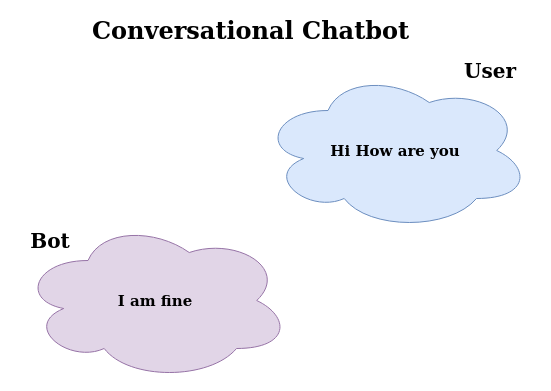Figure 1: Conversational Chatbot

Transformers architecture was introduced in Attention is all you need paper. Similar to CNN for Computer vision, the transformers are for NLP. A simple daily use case one can build using transformers is Conversational Chatbot.

We won’t get into the history of sequence models like LSTMs, RNN, and GRU which were used for similar use cases but just one thing to keep in mind, these models weren’t able to capture long-range dependencies as the passage or text becomes longer and longer.

Transformer architecture consists of an encoder and a decoder network. In the below image, the block on the left side is the encoder (with one multi-head attention) and the block on the right side is the decoder (with two multi-head attention).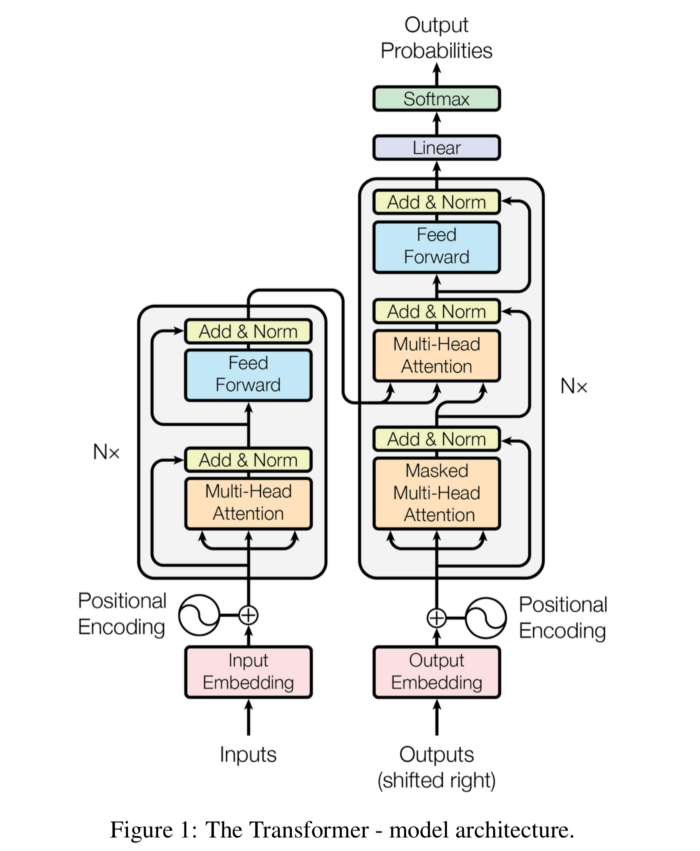Figure 2: Transformer Architecture

First, I will explain the encoder block i.e. from creating input embedding to generating encoded output, and then decoder block starting from passing decoder side input to output probabilities using softmax function.

...

## Encoder Block

Transforming Words into Word Embedding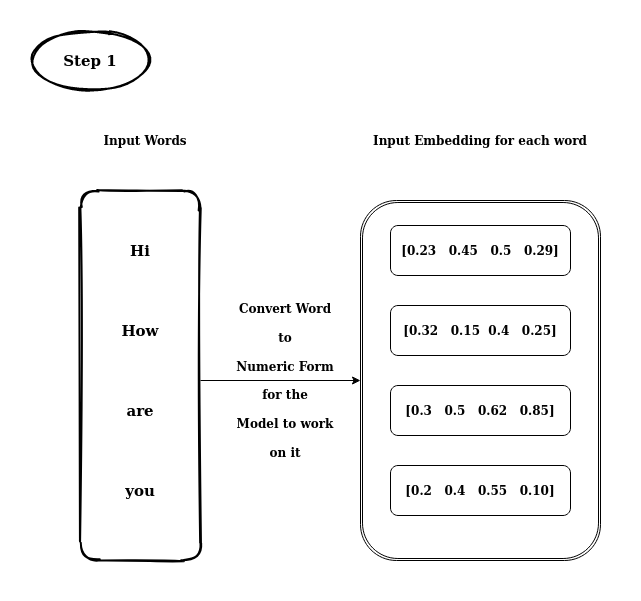Figure 3: Transforming Text to Embedding

### Creating Positional Encoding

Positional encoding is simply a vector generated using a function based on condition. For instance, we can condition that on odd input embedding, we’ll use cos function to generate a position encoding (a vector), and on even input embedding, we’ll use sin function to generate a positional encoding (a vector).

$$PE_{(pos,2i)} \ = \ sin(pos/10000^{2i/dmodel}) \\ PE_{(pos,2i+1)} \ = \ cos(pos/10000^{2i/dmodel})$$Figure 4: Create Positional Encoding

### Adding Positional Encoding and Input EmbeddingFigure 5: Combing Input with Position Encoding

#### Creating Query, Key and Value Vectors

In the last step, we generated Positional Input Embedding. Using this embedding, we create a set of Query, Key, and Value Vectors using Linear Layers. To be clear, for each word we’ll have Q, K, and V vectors.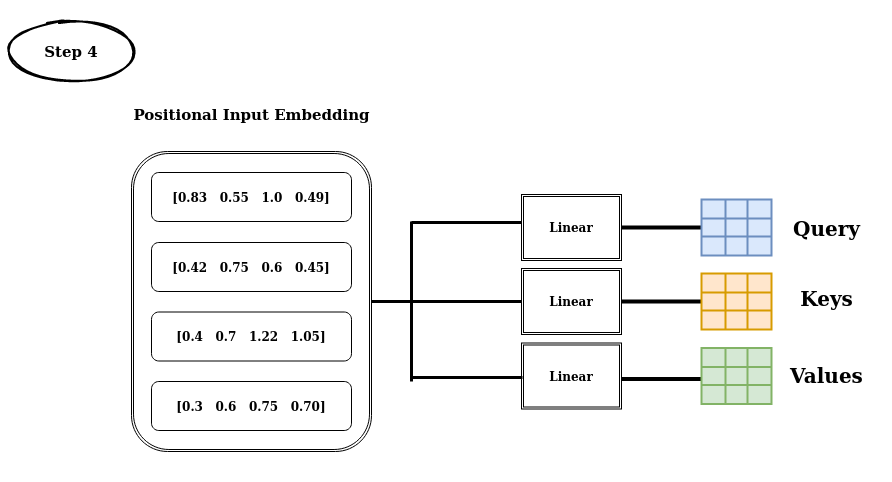Figure 6: Creating Q, K and V

• The best analogy is seen in stack overflow for Q, K and V is of Youtube Search, where the text search of a video is the Query and that words in query are mapped to keys in youtube DB and which in turn brings out values i.e. videos.*

Multi-head attention uses a specific attention mechanism called self-attention. The purpose of self-attention is to associate each word with every other word in the sequence.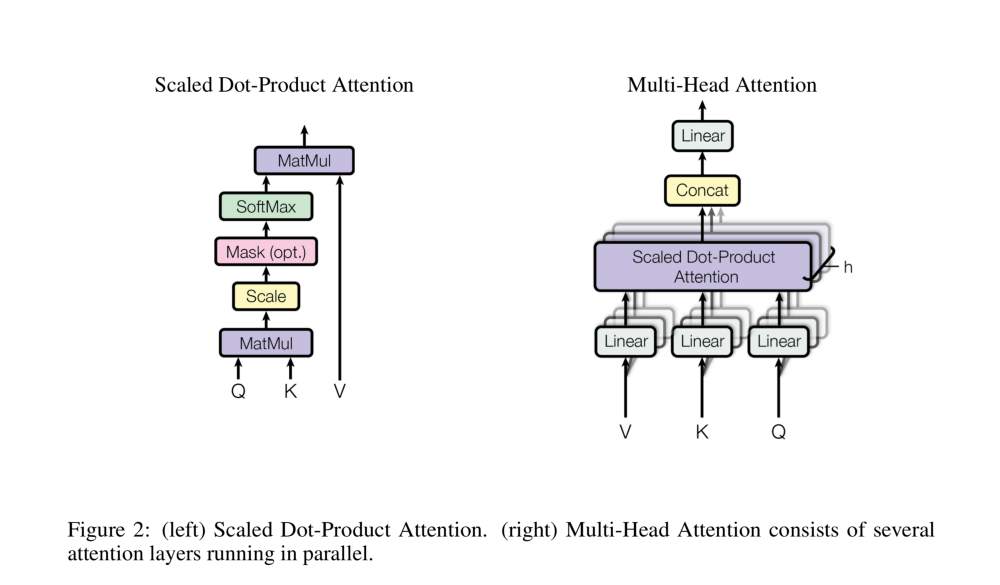Figure 7: Attention Module

In the above image, we can see Mask (opt.) in the attention network because we’ll use masking while decoding and it’s not required in the encoder’s multi-head attention. We’ll discuss masking while exploring the decoder side of the transformer network.

### Dot Product Between Q and V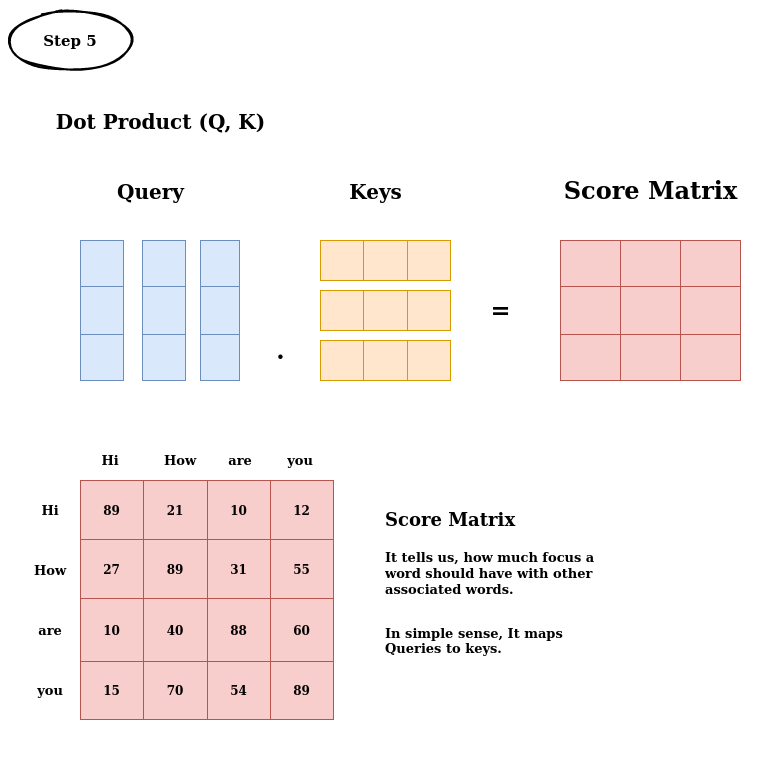Figure 8: Matrix Multiplication Between Query and Keys

### Scaling Down Score Matrix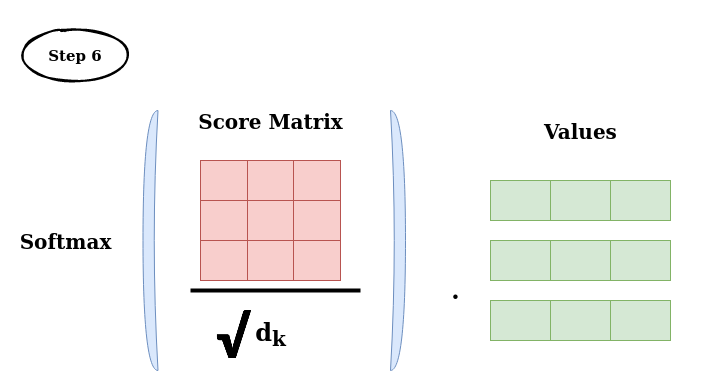Scaling, Softmax and then MatMul with Value

• Score matrix is generated after performing dot product between queries and keys.

• To stabilize the gradients from having gradient explosion, we scale the score matrix by dividing it using √d_k, d_k is the dimension of keys and queries.

• After scaling down the score matrix, we perform a softmax on top of the scaled score matrix to get the probabilities score. This matrix with probability score is called attention weight.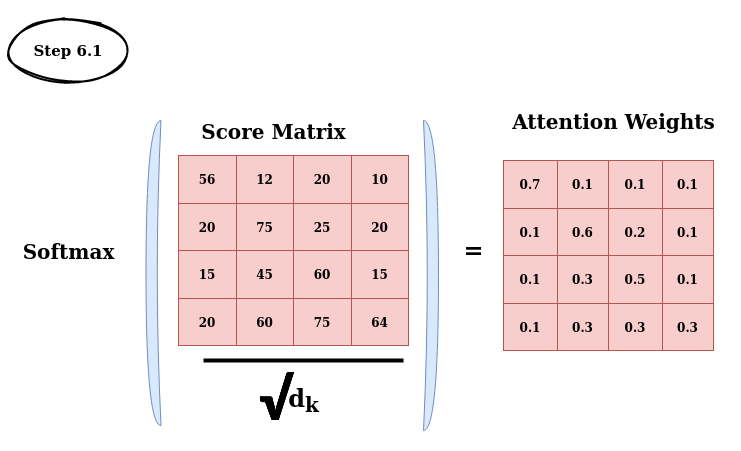Figure 9: Creating Attention Weights

• And after that we perform the dot product between values and attention weights.

• It helps in attending to specific words and omit other words with a lower probability score.

### Drowning Out Irrelevant Words using Attention Weights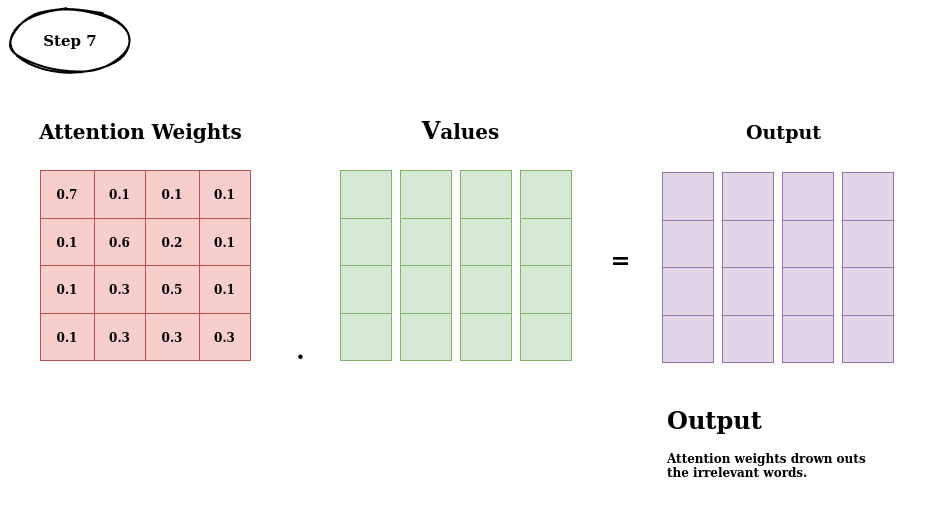Figure 10: Drowning Out Irrelevant Word using Attention Weights

### Feed Forward Neural NetworkFigure 11: Refining results using FFNN

The encoder output we have seen is of one encoder or one single attention block. Next, we’ll see what is multi-head means here.

Now, all the steps we’ve seen under Encoder Block are just Single Head of Multi-Head Attention, to make it multi-head, we copy Q, K, and V vectors across different N heads. Operations after generating Q, K, and V vectors is called self-attention module.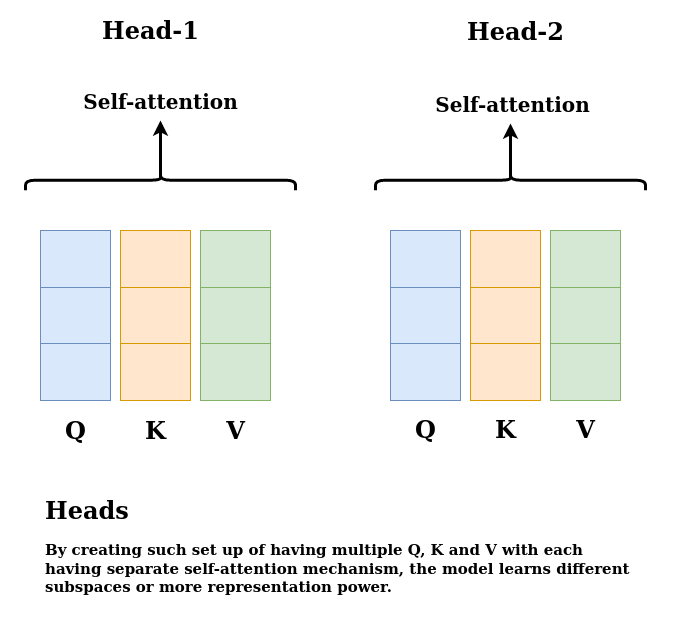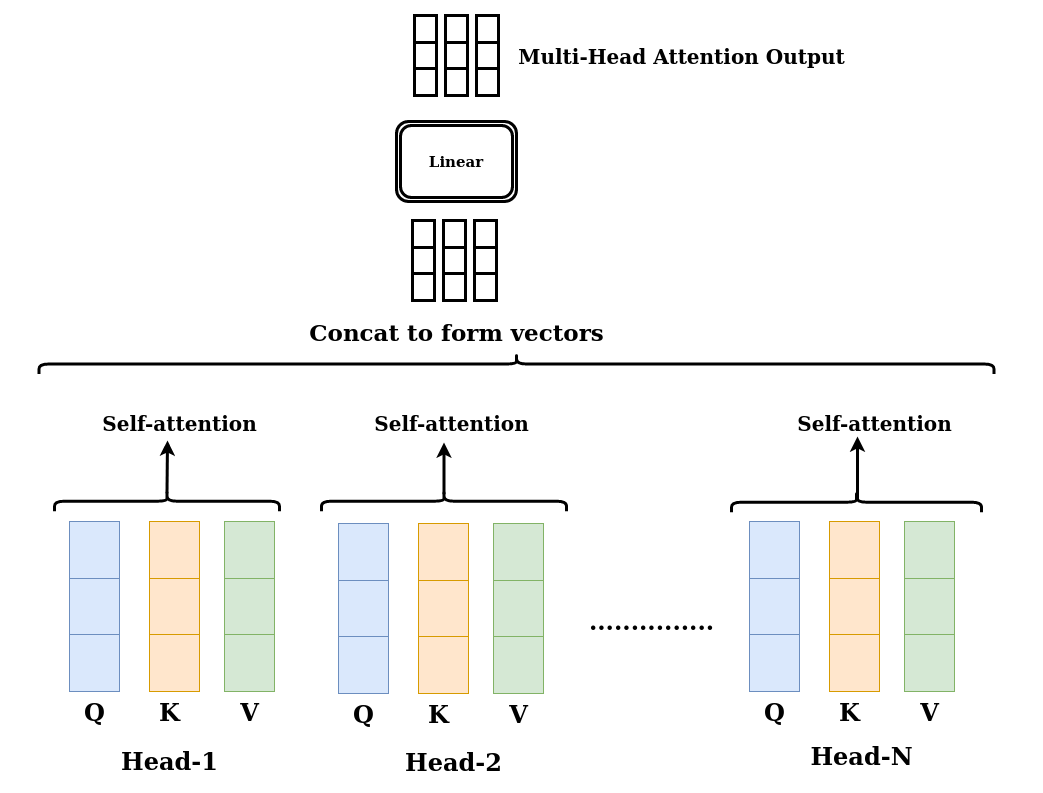### Encoder Output

If we check out the transformer architecture, we see multiple residual layers and x N on both sides of Encoder and Decoder Block, which means multiple Multi-Head Attention each focusing and learning wide representation of the sequences.

Residual layers are used to overcome the degradation problem and vanishing gradient problem. Check out Resnet paper for the same.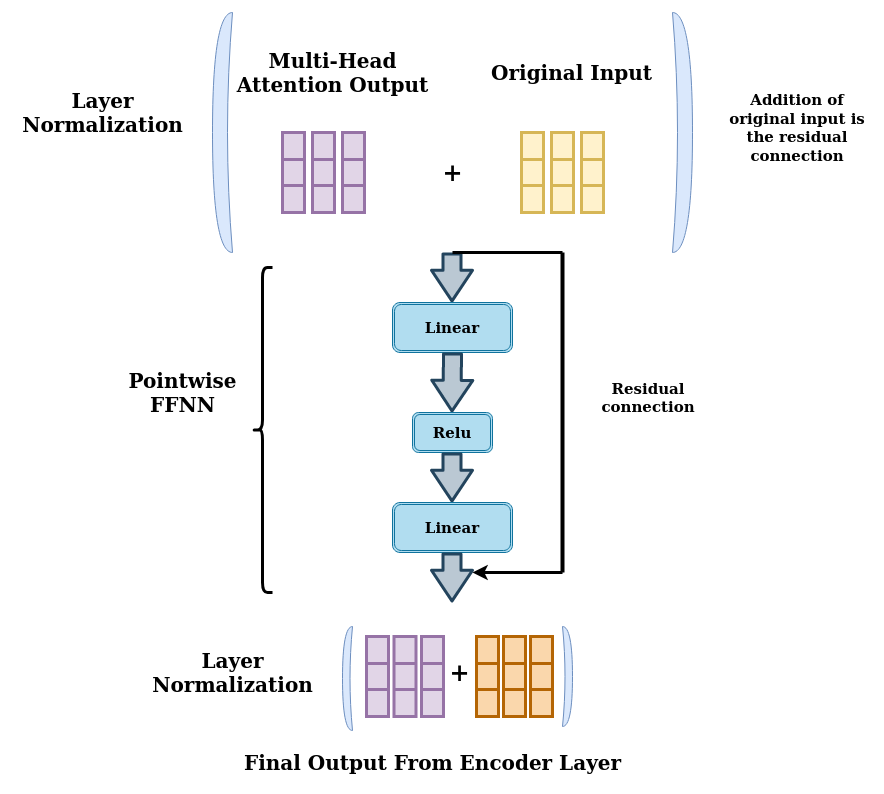Figure 14: Encoder Block Output

In Summary, the Multi-Head Attention Module in the transformer network computes the attention weights for the inputs and produces an output vector with encoded information of how each word should attend to all other words in the sequence.

...

## Decoder Block

In the decoder block, we have two multi-head attention modules. In the bottom masked multi-head attention, we pass in decoder input, and in second multi-head attention, we pass in encoder’s output along with the first Multi-head attention’s output.

Decoder does a similar function as encoder but with only one change, that is Masking. We’ll see down the line what is masking and why it is used.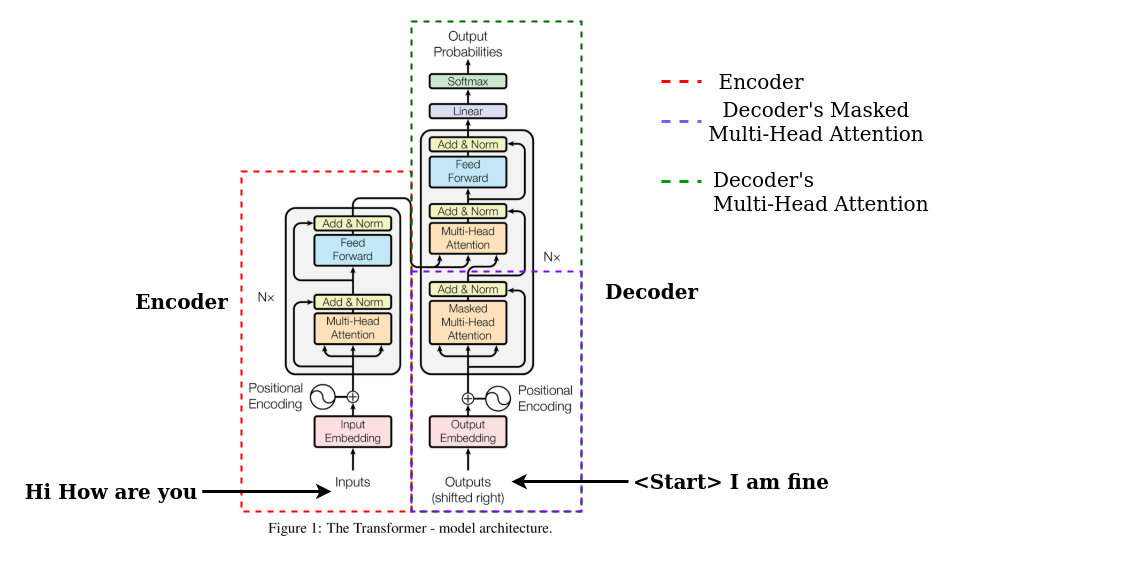Figure 15: Encoder and Decoder Block

It should be noted that decoder is an auto-regressive model meaning it predicts future behavior based on past behavior. Decoder takes in the list of previous output as input along with Encoders output which contains the attention information of input (Hi How are you). The decoder stops decoding once it generates token.

Encoder’s output is considered as Query and Keys of second Multi-Head Attention’s input in the decoder and First masked multi-head attention’s output is considered as the value of second Multi-Head Attention Module.

### Creating Value Vectors

The first step is creating the value vectors using decoder input and generating attention weights.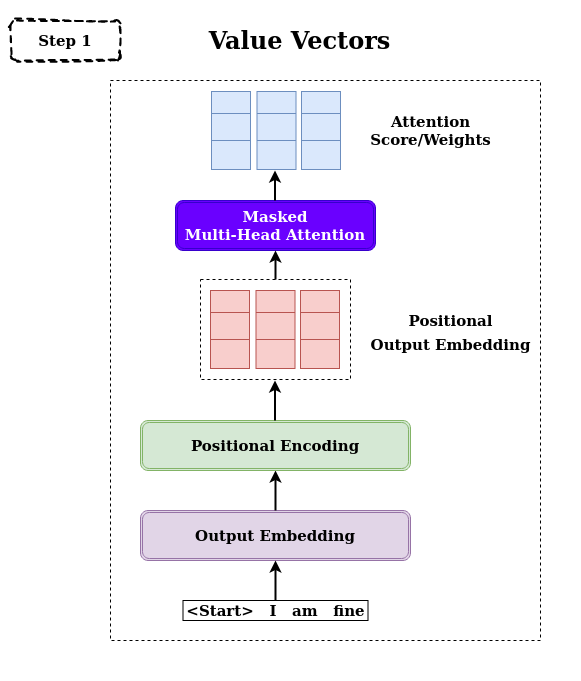Figure 16: Creating Value Vector

Masked Multi-Head Attention generates the sequence word by word we must condition it to prevent it from looking into future tokens.

As said earlier, the decoder is an auto-regressive model and it takes previous inputs to predict future behavior. But now, we have our input I am fine, our decoder shouldn’t see the next input beforehand because the next input is the future input for the decoder to learn.

For instance, while computing the attention score for input word I, the model should not have access to the future word am. Because it is the future word that is generated after. Each word can attend to all the other previous words. To prevent the model from seeing the future input, we create a look-ahead mask.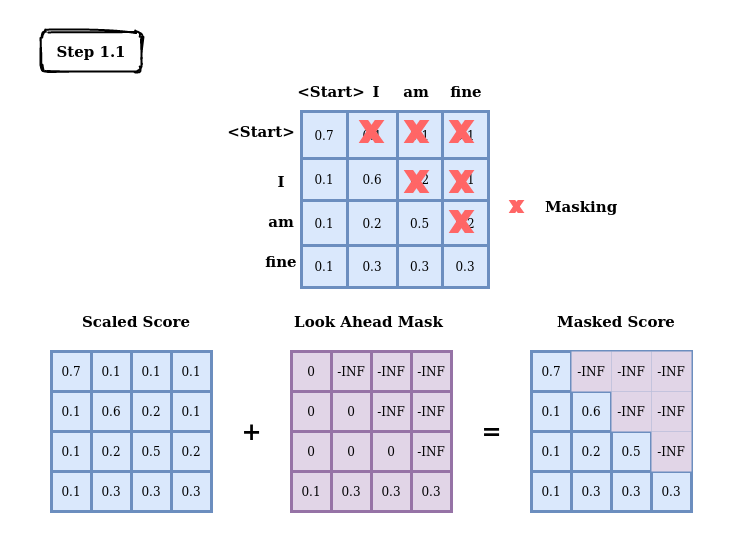### Masking is added before calculating the softmax and after scaling the scoresFigure 18: Softmax on Masked Score

The marked zeros essentially become irrelevant and similarly, this is done on multiple heads and the end vectors are concatenated and passed to the Linear layer for further processing and refining.

In summary, the first Masked Multi-Head Attention creates a masked output vector with information on how the model should attend to the input of the decoder.Figure 19: Decoder’s Multi-Head Attention Output

• Multi-Head attention matches the encoder’s output with decoder output (masked output) allowing the decoder to decide which encoder output is relevant and to focus on.
• Then the output from second multi-head attention is passed through pointwise FFNN for further processing.
• The output of FFNN through Linear Layer, which acts as Classifier Layer
• Each word of vocab has a probability score after going through the softmax function.
• The max. probability is our predicted word. This word is again sent back to lists of decoder inputs.
• This process continues until the decoder generates the token.
• I’ve not mentioned the residual network as drawn in architecture.

The above process is extended again with N head with copies of Q, K, and V making different heads. Each head learns a different proportion of the relationship between the encoder’s output and decoder’s input.

...

If you’ve liked this post, please don’t forget to subscribe to the newsletter.

### Reference

Illustrated Guide to Transformers Neural Network: A step by step explanation

...

Feedback is welcomed 💬